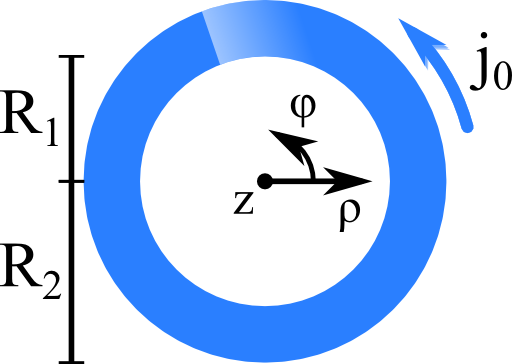## A Cylinder Shell Current and its Magnetic FieldAmpère's law is hard to solve in general. What if certain symmetries of a current distribution are present? We may find much simpler differential equations which might be integrated directly. Find out what to do in rotational and translational symmetry!

## Problem StatementLet us assume a "cylinder shell current distribution" of the following form:$\begin{eqnarray*} \mathbf{j}\left(\mathbf{r}\right)&=&\begin{cases} j_{0}\mathbf{e}_{\varphi} & \rho\in\left[R_{1},R_{2}\right]\\0 & \text{else}\end{cases}\ . \end{eqnarray*}$This current distribution is rotationally symmetric which means that it does not vary in $$\varphi$$. Furthermore, the current distribution is infinitely extended in the $$z$$-direction. This is called a translational symmetry.

Use Ampère's law to determine the magnetic field $$\mathbf{H}\left(\mathbf{r}\right)$$ everywhere for the given current distribution.

If you found a solution for the cylinder shell current distribution, how would you determine the magnetic field of a more general rotationally and translationally invariant current distribution, i.e. $\mathbf{j}\left(\mathbf{r}\right) = j\left(\rho\right)\mathbf{e}_{\varphi}\ ?$

## Hints

Use cylindrical coordinates - the current distribution has only one component then.

Remember that the curl of a vector field in this coordinate system is given by$\begin{eqnarray*} \nabla\times\mathbf{F}\left(\mathbf{r}\right)&=&\left(\frac{1}{\rho}\partial_{\varphi}F_{z}\left(\mathbf{r}\right)-\partial_{z}F_{\varphi}\left(\mathbf{r}\right)\right)\mathbf{e}_{\rho}+\left(\partial_{z}F_{\rho}\left(\mathbf{r}\right)-\partial_{\rho}F_{z}\left(\mathbf{r}\right)\right)\mathbf{e}_{\varphi}\\&&+\frac{1}{\rho}\left(\partial_{\rho}\left[\rho F_{\varphi}\left(\mathbf{r}\right)\right]-\partial_{\varphi}F_{\rho}\left(\mathbf{r}\right)\right)\mathbf{e}_{z}\ .\end{eqnarray*}$

## Show Solution

Ampère's law relates magnetic field and current distribution,$\nabla\times\mathbf{H}\left(\mathbf{r}\right) = \mathbf{j}\left(\mathbf{r}\right)\ .$The (too) straight forward approach to find the solution would be to take the vector potential and solve its integral equaton. Such an approach would be extremely involved. Let us find a much simpler approach, at first for the "cylinder shell current distribution".

## The Magnetic Field of the "Cylinder Shell Current Distribution"

However, if we look at the current distribution, we see that the curl of the magnetic field can only have an angular component. In cylindrical coordinates we find that$\partial_{z}H_{\rho}\left(\mathbf{r}\right)-\partial_{\rho}H_{z}\left(\mathbf{r}\right) = \begin{cases}j_{0} & \rho\in\left[R_{1},R_{2}\right]\\0 & \text{else}\end{cases}$ must hold.

Let us now employ the two symmetries of the current distribution which is the most efficient way to determine the magnetic field.  Since there is no change of the current distribution in $$z$$-direction (cause), the corresponding partial derivative of the magnetic field (effect) must vanish. Furthermore, the magnetic field cannot depend on $$\varphi$$ for the same reason. So, we have to solve the equation$-\frac{dH_{z}\left(\rho\right)}{d\rho} = \begin{cases}j_{0} & \rho\in\left[R_{1},R_{2}\right]\\0 & \text{else}\end{cases}\ .$We have achieved here that we could transform a partial differential equation into an ordinary differential equation as we did for example in The Electric Field and Potential of a Homogeneously Charged Sphere.

We can determine the solution upon integration of both sides of the latter equation. For the left hand “field” side we find$-\int_{0}^{\rho}\frac{dH_{z}\left(\rho^{\prime}\right)}{d\rho^{\prime}}d\rho^{\prime} = H_{z}\left(0\right)-H_{z}\left(\rho\right)\ .$For the current distribution we have, formally written with the use of the Heaviside $$\Theta$$-distribution$j_{0}\int_{0}^{\rho}\Theta\left(R_{1}-\rho^{\prime}\right)\Theta\left(\rho^{\prime}-R_{2}\right)d\rho^{\prime} = j_{0}\begin{cases}0 & \rho<R_{1}\\\rho-R_{1} & \rho\in\left[R_{1},R_{2}\right]\\R_{2}-R_{1} & \rho>R_{2}\end{cases}\ .$Now we still have a little problem: Is the field really vanishing at $$\rho=0$$? From our experience with coils we would have thought that the field has to be strongest here. Also, the magnetic field is constant for $$\rho\rightarrow\infty$$ which is basically unphysical. Fortunately, we can still define $$H_{z}\left(0\right)$$ such that the magnetic field vanishes at infinity. Under this condition we can see that $H_{z}\left(0\right) = j_{0}\left(R_{2}-R_{1}\right)\ .$ Then, finally, the magnetic field is given by$\begin{eqnarray*} \mathbf{H}\left(\mathbf{r}\right)&=&j_{0}\left(R_{2}-R_{1}-\begin{cases}0 & \rho<R_{1}\\\rho-R_{1} & \rho\in\left[R_{1},R_{2}\right]\\R_{2}-R_{1} & \rho>R_{2}\end{cases}\right)\mathbf{e}_{z}\\&=&j_{0}\mathbf{e}_{z}\begin{cases}R_{2}-R_{1} & \rho<R_{1}\\R_{2}-\rho & \rho\in\left[R_{1},R_{2}\right]\\0 & \rho>R_{2}\end{cases}\ . \end{eqnarray*}$Interestingly, the magnetic field outside of the current distribution vanishes!

## General Rotationally and Translationally invariant Current Distributions

We have found the magnetic field for a specific rotationally symmetric current distribution. Now it is also clear how we would find the solution for a more general current distribution $$j\left(\rho\right)\mathbf{e}_{\rho}$$ - we just have to leave out the explicit integration before and write$H_{z}\left(\rho\right) = H_{z}\left(0\right)-\int_{0}^{\rho}j\left(\rho^{\prime}\right)d\rho^{\prime}\ .$In addition, to be consistent with a vanishing field at infinity, we know that$H_{z}\left(0\right) = \lim_{\rho\rightarrow\infty}\int_{0}^{\rho}j\left(\rho^{\prime}\right)d\rho^{\prime}\ .$Yet again we have seen how useful symmetries can be!